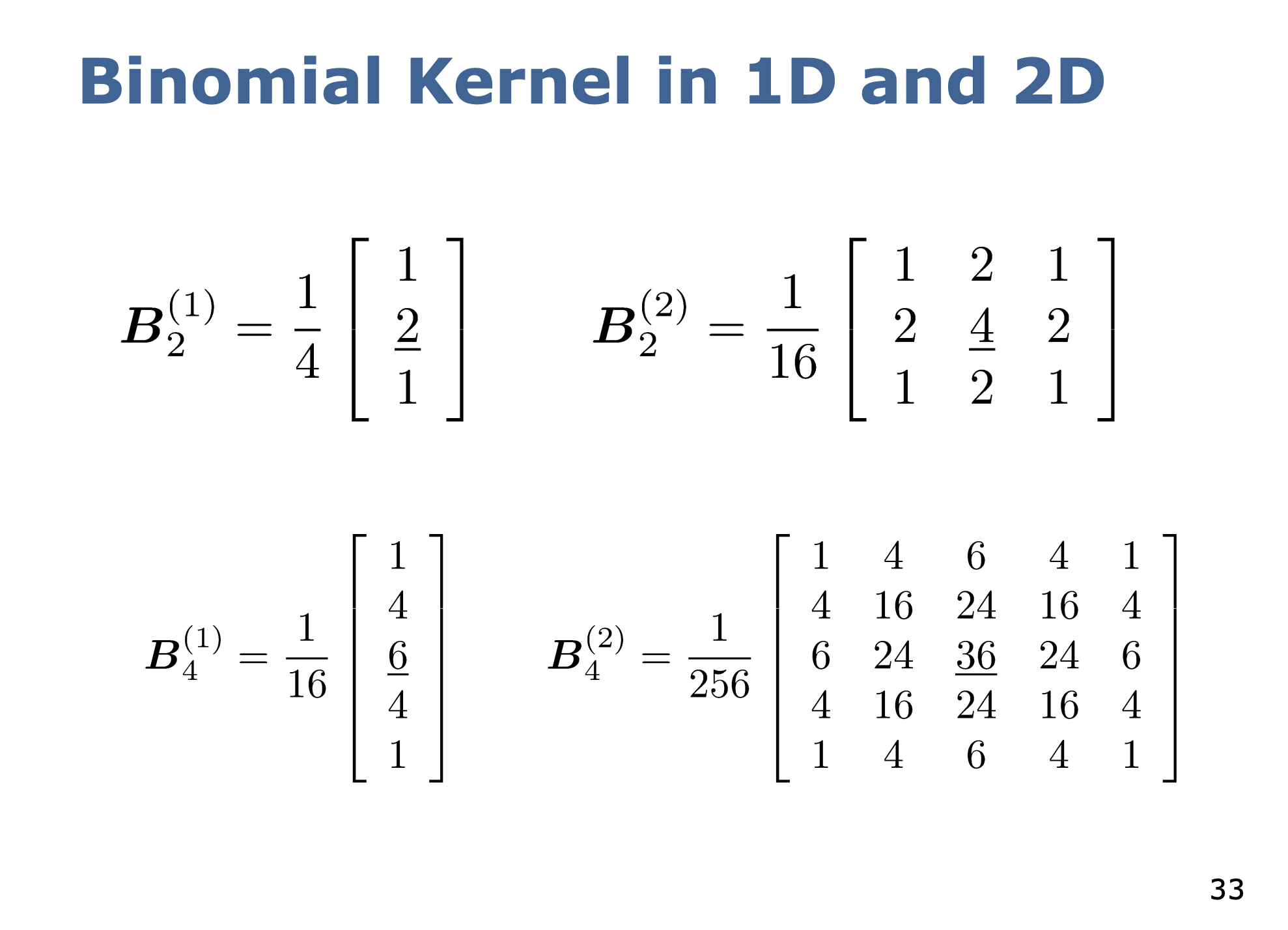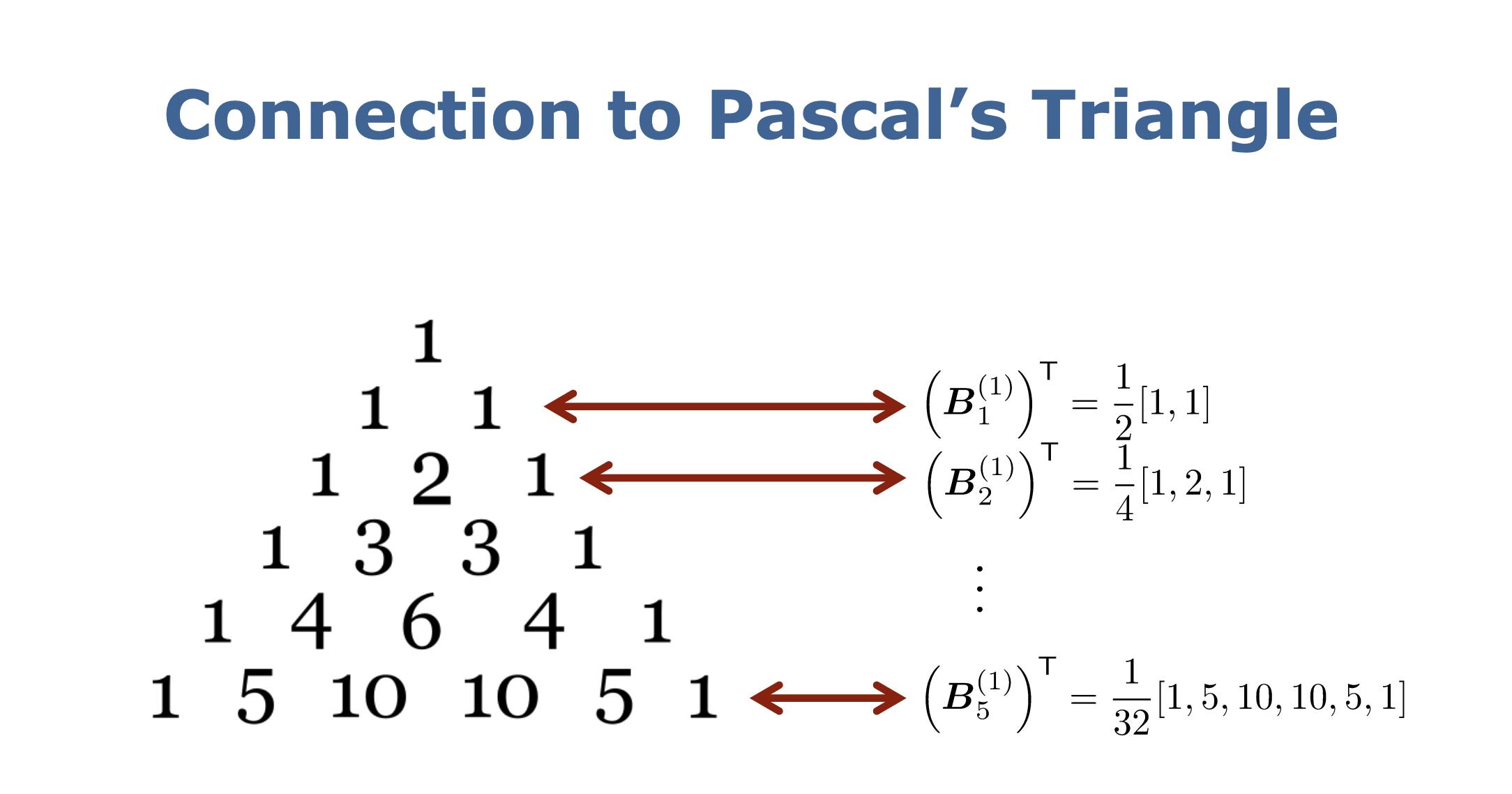# Binomial Filter §

Difference with Gaussian Filter?

Gaussian is perfectly radial and isotropic, while a binomial filter has more “rectangular” response, filtering less of the diagonals.

A binomial filter approximates a gaussian distribution.Binomial Filter

• Performs a smoothing
• Smoothing using an approximation of a Gaussian as the kernel function
• Discrete approximation due to pixels
• The decay of the weights approximates a Gaussian using the coefficients of a Binomial distribution
• Elements of the Pascal’s triangleSo when you apply a gaussian blur, it’s just applying the Binomial Kernel.

Degree of smoothing

• Box filter offers a stronger smoothing than the binomial filter.